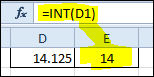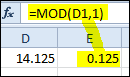# Extracting Integers and Fractions in Microsoft Excel

Sometimes you need to extract the integer portion of a number. Sometimes the fractional part. Sometimes both. Excel makes it easy to get the integer and somewhat harder to get the fraction. If you just want the answer, skip to the technical details.

### The Integer Part: Excel INT Function

What could be easier than the Excel INT function? I mean INT almost screams INTEGER. So the name is intuitive. You almost “know” what it’s going to do, even if you haven’t used it before.

With only one argument, it’s execution is even simpler. Just feed it a number and out pops the integer portion.

Below you can see I have the number 14.125 in cell D1 and the formula =INT(D1) returns the integer 14 in cell E1.Date/Time Tip: A practical application for the INT function is to extract the date value from a date/time number. An example is a date/time value like 2/14/2013 9:04 AM in cell A2 and you only want the date. Using the formula =INT(A2) will strip out the time and leave the date 2/14/2013.

### The Fractional Part

Here is where I would like some simplification. I mean, if we have the INT function for extracting an integer, you would think that there would be a FRAC function, or a simple name like that, to extract the fractional part.

If there was a FRAC function, you could also imagine that it would have only one argument. Just feed it a number and out pops the fraction. Simple. Elegant. Intuitive. No thought required. But, I digress.

To extract the fractional part of a number we can use the MOD function, which has not one, but two arguments. The reason it has two arguments is because it does more than extracting the fractional part. Forget about that.

Here is what you need to know about the MOD function and how to extract the fractional part of a number. The second argument of the MOD function is 1. Remember that. To reiterate, 1 is the second argument.

The first argument of the MOD function is the number. Shown below, you can see the number 14.125 in cell D1. The formula =MOD(D1,1) returns the fractional part 0.125 in cell E1.Date/Time Tip: A handy way to extract a time value from a date/time number is to use the MOD function. Per our previous example, the date/time number 2/14/2013 9:04 AM in cell A2, the formula =MOD(A2,1) will return 9:04 AM.

#### Technical Details

Assume you have a number in cell A1.

To extract the integer value, use the formula:
=INT(A1)

To extract the fractional value, use the formula:
=MOD(A1,1)## 3 thoughts on “Extracting Integers and Fractions in Microsoft Excel”

1.James Clarkson

Hello Gregory.

The solutions you propose will work well for positive values, but if you test them with negatives, you might find the answers surprising.

A safer solution for all values would be…
Integer value: =TRUNC(A1)
Fractional value: A1-TRUNC(A1)

1.Gregory

Quite frankly, it never entered my mind that negative numbers would be used. And you are absolutely correct that the TRUNC function is a better way to extract integers. From the TRUNC function, the following tells the story.

TRUNC and INT are similar in that both return integers. TRUNC removes the fractional part of the number. INT rounds numbers down to the nearest integer based on the value of the fractional part of the number. INT and TRUNC are different only when using negative numbers: =TRUNC(-4.3) returns -4, but =INT(-4.3) returns -5 because -5 is the lower number.

Thanks for the comment.

2.James Clarkson

No worries Gregory. However, in finance negatives are common, as sign is used to distinguish between inflows vs outflows, revenue vs expenses, debits vs credits etc, so sign has to be approached carefully and all solutions need checking to avoid gotchas with negatives.